omG8HI9LSKFW6lhCj8prTs0Z6lUhdOT9Jhi1Sf4m

# What is Ohm's Law? Theory, Formula, and Ohms Law Calculator

In the previous post we have talked about voltage, current, and power in an electric circuit. In order to determine the values for given elements, we need to understand some fundamental laws for electric circuits. These two : Ohm's Law and Kirchhoff's Laws are the fundamental laws for other circuit analysis technique that have been discovered and used for years.

## Ohms Law Wheel

Ohm's Law shows the relationship between Voltage (V or E), Current (I), and Resistance (R). Thus, we add the Joule's law to perfect the ohms law wheel. Joule's law states that power is the multiplication of voltage and current. As a result, the combination of these two will provide us with 12 fomulas with 2 known variables. Therefore, we get the ohm's law wheel below along with their measurement units.

In this section, we will discuss some techniques which will help us greatly for analyzing electric circuits. But, we will limit the analysis technique for resistive load only to make it easier to understand. After some practices we will try to implement it to another types of load.

## What is Ohm's Law

According to American English Dictionary, Ohm's Law is the proportionality of current in dc circuit to applied voltage and inversely proportional to resistance. Georg Ohm, a German physicist is the inventor of this law in 1827.

Each material has its own characteristic of resisting the electric charge flow. The physical ability to resist current is known as resistance and is represented with the symbol R.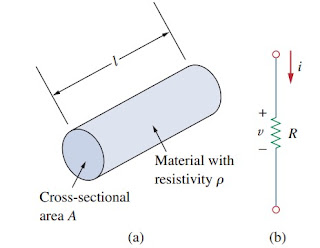Figure 1. Resistance
The resistance of material with a uniform cross-sectional area A depends on A and the length l, as can be seen in Figure.(1a). Thus, the resistance mathematical form can be represented below :(1)
where ρ is resistivity of material (ohm-meters). Good conductors such as copper and aluminium have low resistivities, while insulators such as mica and paper have high resistivities. Therefore, if you want to know about the resistivities of common material you can find it in the internet or simply click here : material resistivities which divide the material into conductors, insulators, and semiconductors.

Resistor is usually made from metallic alloys and carbon compounds to fulfill the current-resisting behaviour. The circuit symbol can be seen in Figure.(1b), where the R stands for resistance of the resistor. Resistor is the simplest passive element.
The relationship between current and voltage to resistor is known as Ohm's law, credited to Georg Simon Ohm (1787-1854) written in :
Ohm's law states that the voltage v across a resistor is directly proportional to the current i flowing through the resistor.
Hence,(2)

## Ohms Law Formula

Ohm defined the constant of proportionality for a resistor to be the resistance, R.
Hence, the Equation.(2) becomes(3)
which is mathematical equation of Ohm's law. Thus, R in Equation.(3) is measured in ohms or Ω.
So that,
The resistance R of an element denotes its ability to resist the flow of electric current, measured in ohms (Ω).
We can deduce the equation to(4)
so that
1 Ω = 1 V/A
In order to successfully implement the Ohm's law we need to pay attention to voltage polarity or current flow direction.

The value of R varies from zero to infinity. Hence, it is important to take note two extreme possible values of R. An element with the value R = 0 in Figure.(2a) is a short circuit.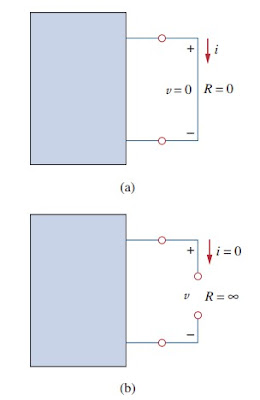Figure 2. Short circuit and open circuit
So that,(5)
indicating that the voltage is zero but the current could be any values. In other words, short circuit is usually assumed by connecting wire that perfect conductor. Hence.
A short circuit is a circuit element with resistance approaching zero.
In opposite, an element with R = ∞ is open circuit as can be seen in Figure.(2b). For an open circuit,(6)
indicating that the current is zero through the voltage could be any values. Hence,
An open circuit is a circuit element with resistance approaching infinity.
A resistor can be either fixed or variable. But, it has fixed value which means the value remains constant. Figure.(3) shows the two common types of fixed resistors (wirewound and composition). The Figure.(3a) is wirewound type has smaller resistance with larger power treshold while the Figure.(3b) is composition type, has higher resistance with smaller power treshold.Figure 3. Fixed resistors
Variable resistors have adjustable resistance and its symbol can be seen in Figure.(4a). A common variable resistor is also known as potentiometer or pot for short, its symbol is shown in Figure.(4b).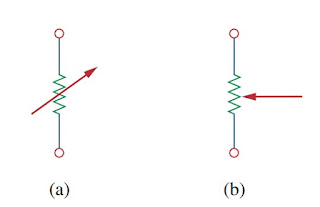Figure 4. Variable resistor
The pot is three-terminal element with sliding contact or wiper. Using the sliding contact will change the resistance. Similarly, variable resistor also has type of wirewound and composition as can be seen in Figure.(5a) for composition and Figure.(5b) for slider pot.Fig.5 Potentiometer
Not all resistors obey Ohm's law. But, a resistor that obeys Ohm's law is called a linear resistor. It has constant resistance. Hence, its current-voltage characteristic can be seen in Figure.(6a) : the i-v graph is straight line passing the origin. An nonlinear resistor does not obey Ohm's law, its resistance varies with current and its i-v characteristic can be seen in Figure.(6b). The example of nonlinear resistance are light bulb and diode.Figure 6. Resistor i-v characteristic
Another useful quantity in electric circuit analysis is reciprocal of resistance R, known as conductance and the symbol is G :(7)
Conductance measures how well an element will conduct electric current and its unit is mho (ohm spelled backwards) or reciprocal ohm with the symbol ℧, an inverted omega. In this blog we will use siemes (S) over mho, as SI unit of conductance :(8)
Hence,
Conductance is the ability of an element to conduct electric current; measured in mhos (℧) or siemens (S)
Same resistance can be expressed in ohms or siemes, for example 10 Ω is the same as 0.1 S. Looking from Equation.(7) we can write :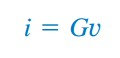(9)
The power dissipated by a resistor can be expressed using R with Equation.(3),(10)
The power dissipated by a resistor can be expressed using G,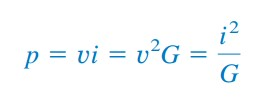(11)

## Ohm's Law Examples

1.An electric iron draws 2 A at 120 V. Calculate its resistance.
Solution :
Using Ohm's law :

2.According to circuit below, calculate the current i, the conductance G, and the power p.

Solution :
The current is :
The conductance is :
The power is :

## Ohms Law Calculator

If you have understood the basic principle of Ohms Law Formula, you can see the conversion below to shorten your work time on it.

 Enter two known values and press Solve to calculate unknowns. Volts (Volts) Resistance (Ohms) Current (Amps) Power (Watts)

Untuk Bahasa Indonesia baca Hukum Ohm
Related Posts
SHARE